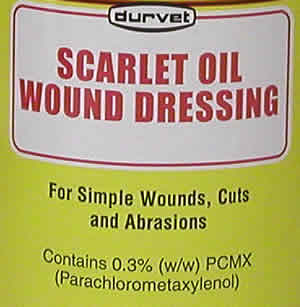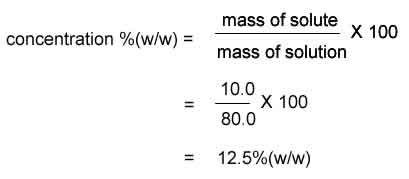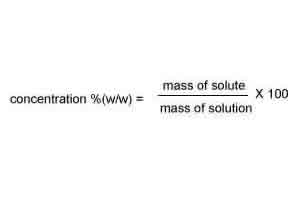Concentration(w/w) The concentration of a solution indicates the relative amount of solute and solvent present. A concentrated solution has a high ratio of solute to solvent. There are many ways to express the concentration of a solution. They vary only in the units that are used but always express the amount of solute in a given volume of solution. The label on the right states that the solution contains 0.3% (w/w) PCMX, where (w/w) is weight per weight. Ok, what dose this tell us? The label 0.3%(w/w) indicates that 0.3 g of PCMX is present for every 100 grams of solution. This is known as percentage by mass and gives the mass of the solute present in 100 grams of solution.80.0 g of salt solution contains 10.0 g of salt. What is the concentration (w/w) of the salt solution?120.0 grams of a sugar solution contains 20.0 grams of sugar. What is the concentration (w/w) of this solution? SolutionWhat mass of a 5.0% (w/w) hydrochloric acid solution will contain exactly 0.50 grams of acid? Solution 200.0 grams of a 15.0% (w/w) hydrochloric acid solution will contain what mass of acid? Solution